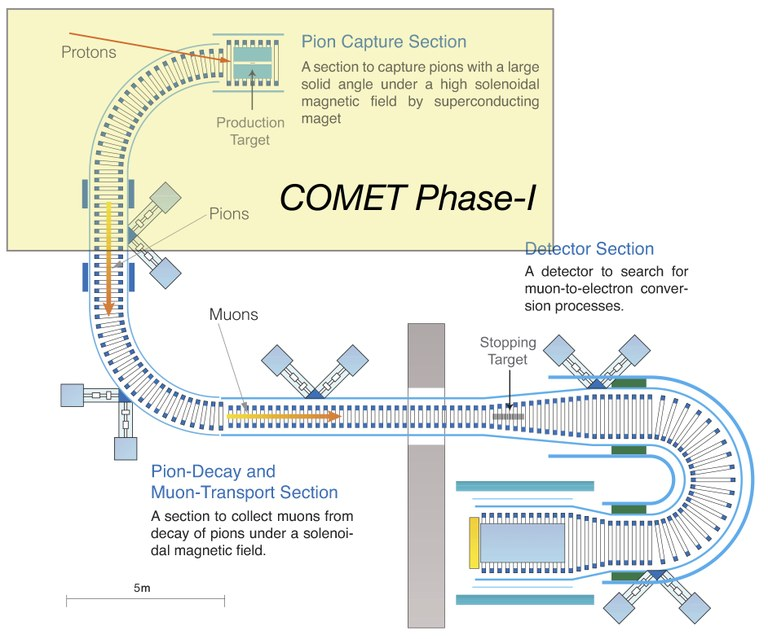# Introduction

Brief introduction of COMET experiment

COMET searches for coherent neutrino-less conversion of a muon* to an electron, μ- + N(A,Z) → e- + N(A,Z), in muonic atoms (μ-e conversion) at a 90% confidence-level sensitivity of B(μ- + Al → e- + Al) <10-16. The target sensitivity is a factor of about 10,000 better than the present published limit of B(μ- + Au → e- + Au) < 7×10-13. This experiment would provide a very large window on new physics beyond the Standard Model.* Muon(µ) is one of elementary particles in the standard model of the particle physics, which is categorised as the 2nd generation of charged leptons.